# Coin-Counting Clock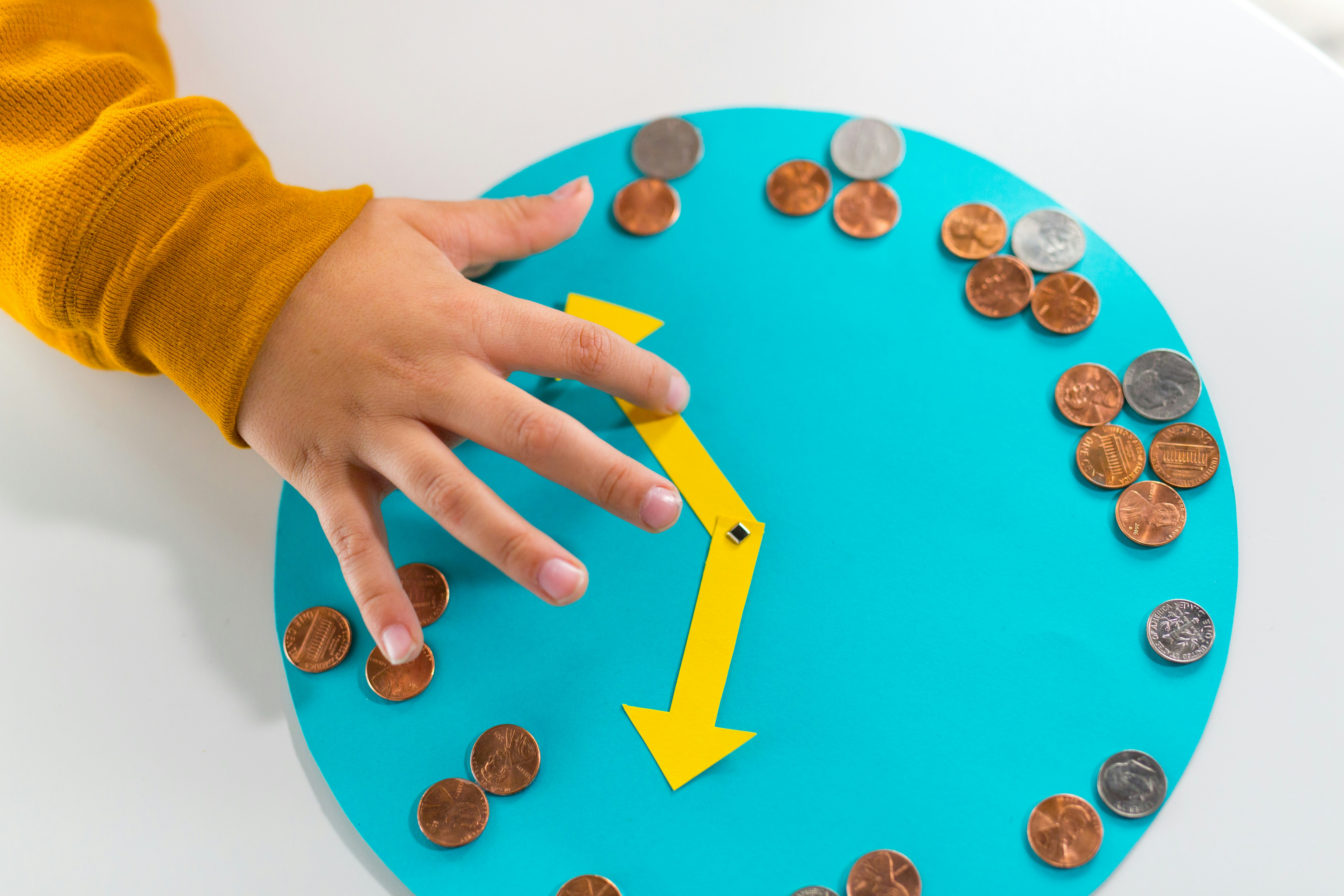Kids can learn to tell time and count money with a fun clock.

Activity Time: 30 – 45 minutes (Plus, 30 minutes of drying time)

STEAM Subjects: Math (Telling Time, Currency), Art

Supplies:

• Dinner plate
• 12x12” sheet of paper
• Small scrap sheet of paper (approx. 4X4 inches)
• Loose change (21 quarters, 5 nickels, and 3 dimes)
• Elmer’s Extra Strength Glue Stick
• Scissors
• Ruler
• Pencil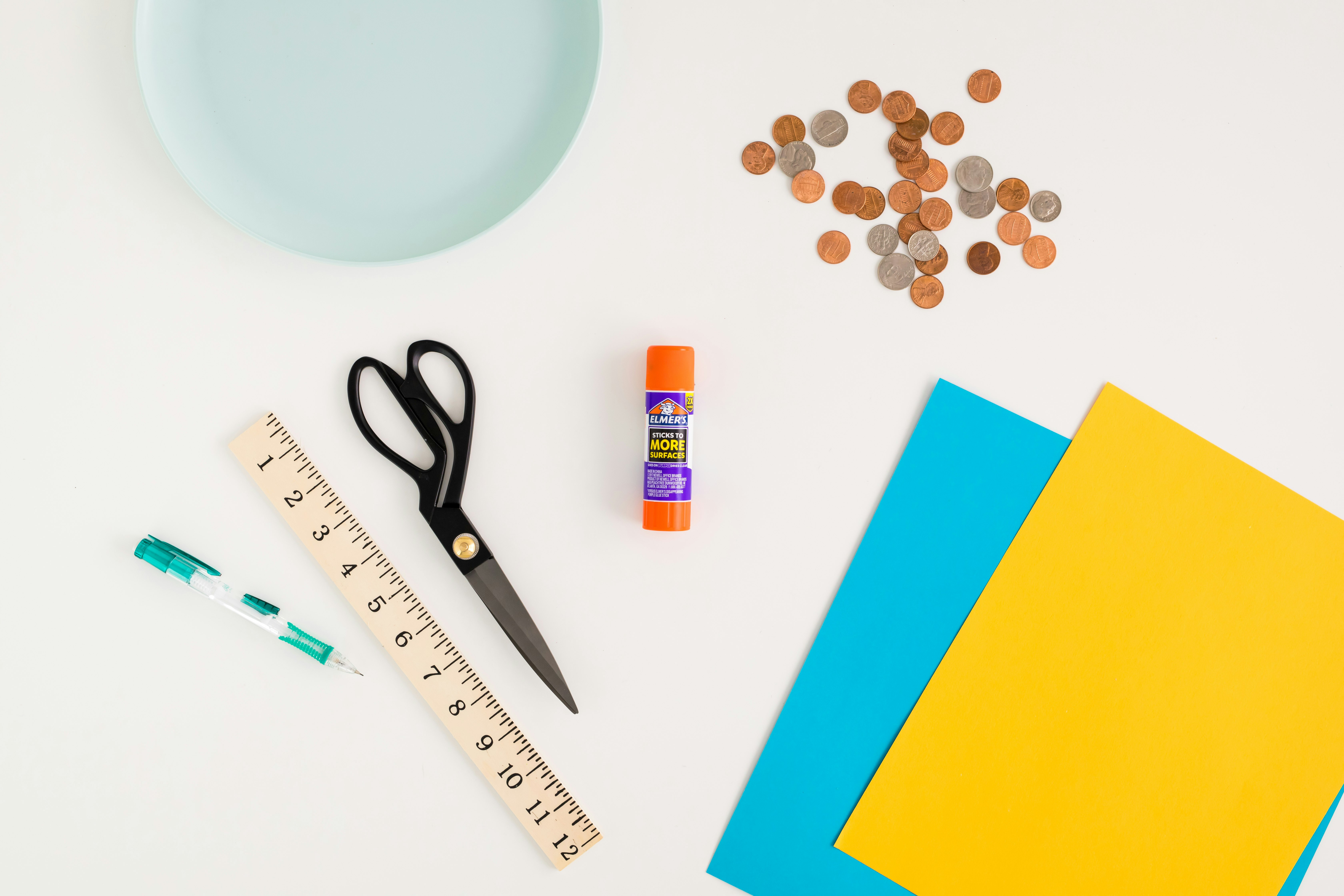Instructions:

• Use the dinner plate to trace a circle onto the 12x12 piece of paper. Use scissors to cut out the circle.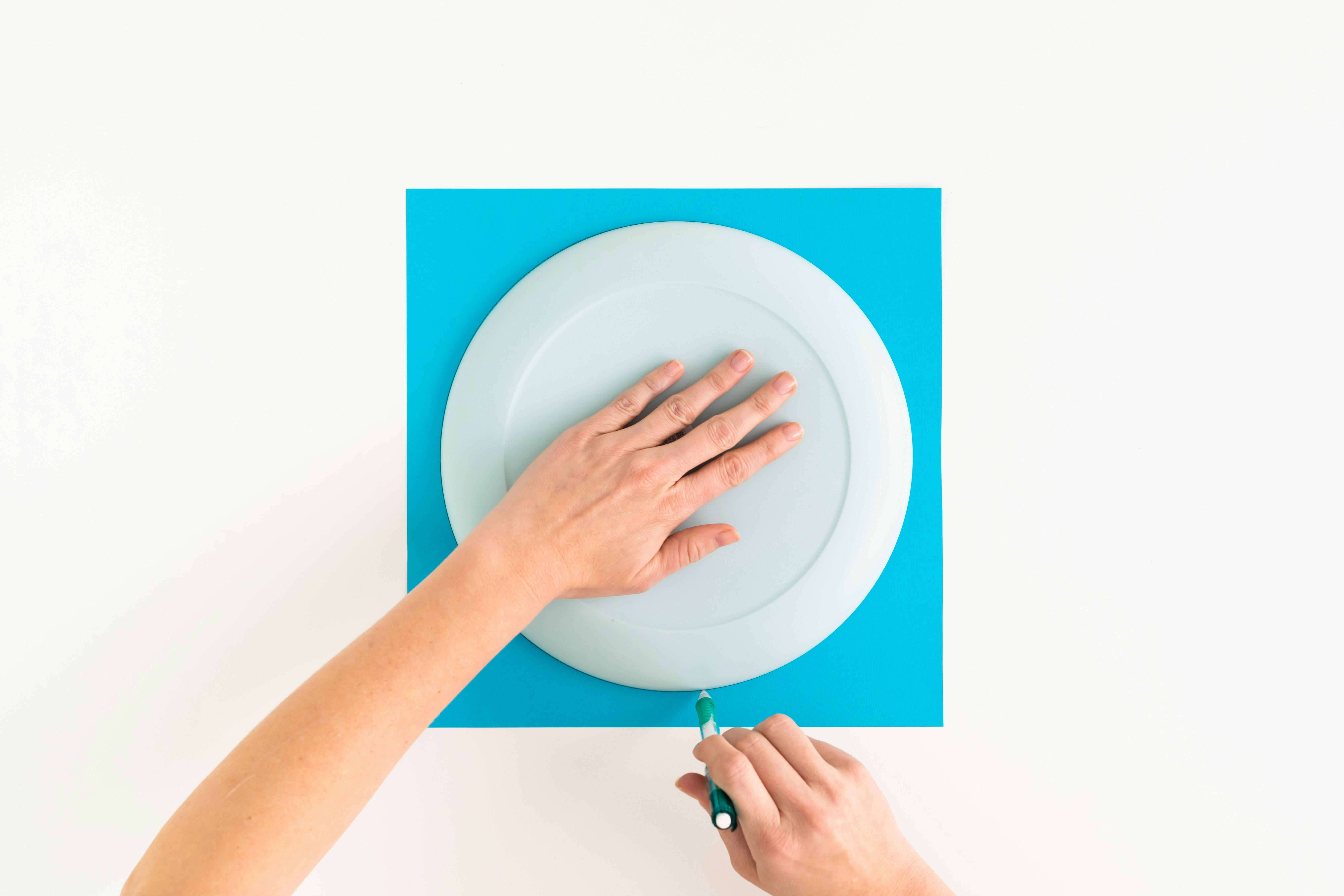• Draw and cut out arrows from a scrap sheet of paper.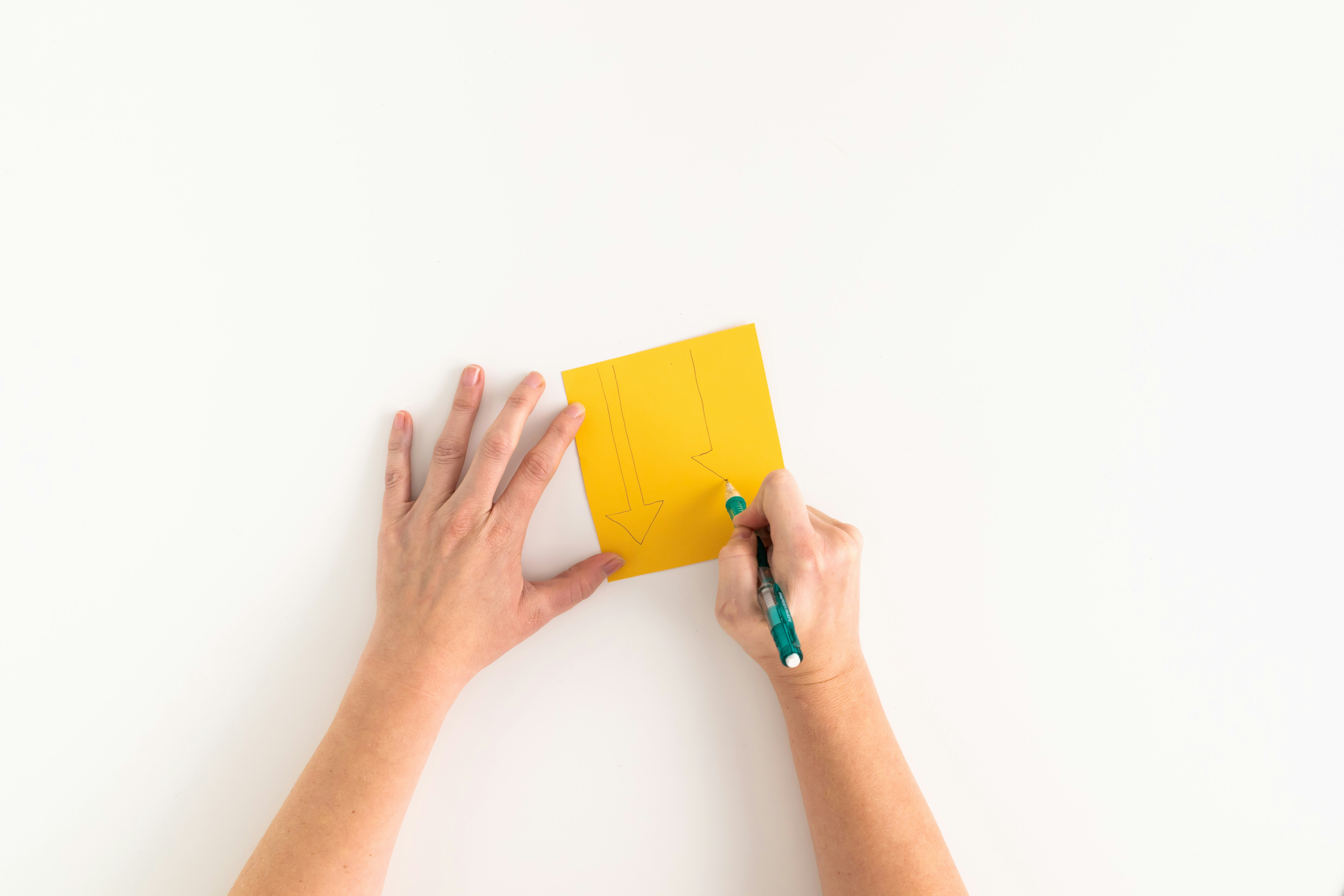• Attach the arrows to the centre of the circle with a brad.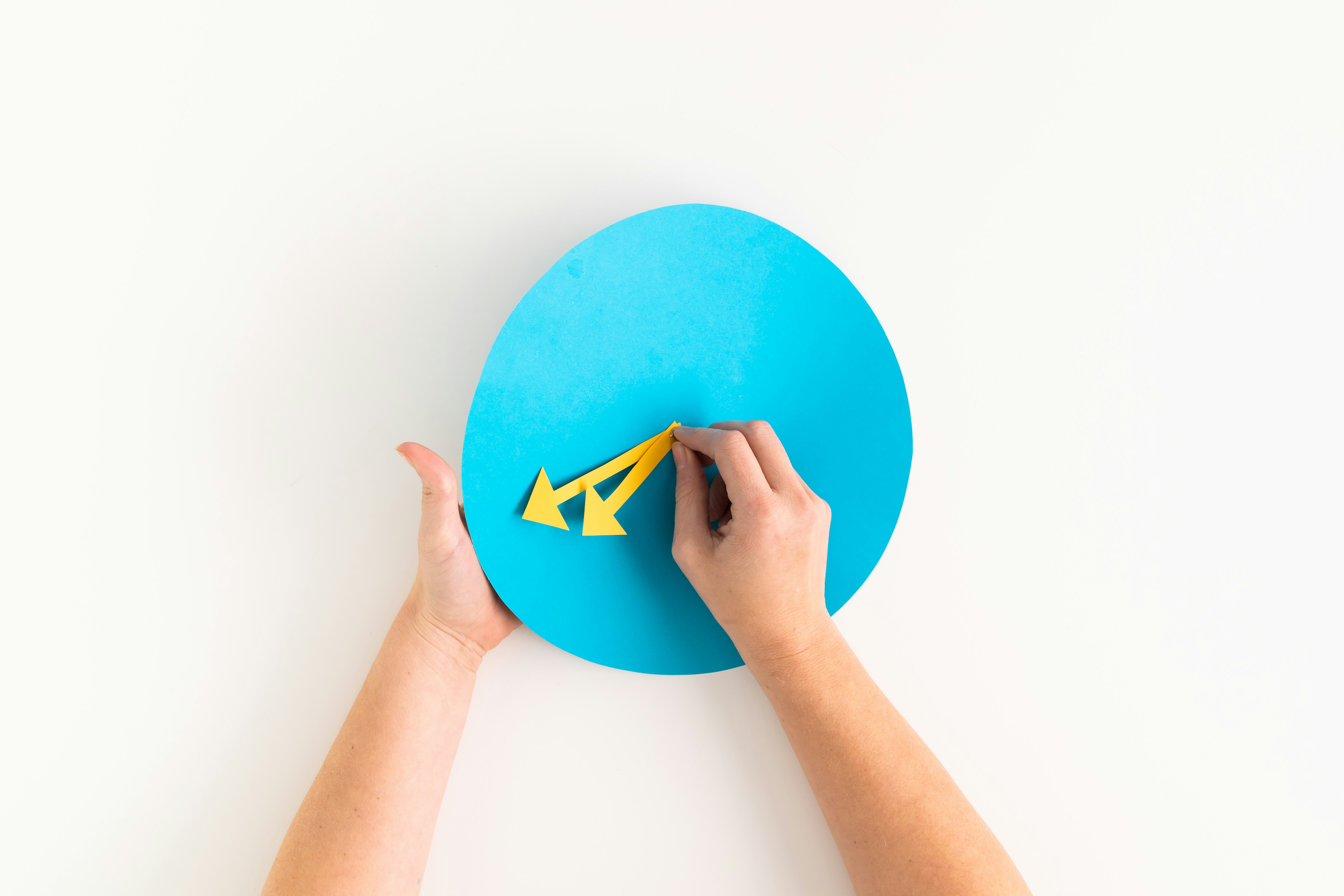• Count out the coins, using the quarters, nickels, and dimes to represent numbers on the clock. For example, one o’clock is represented by one quarter. Six o’clock is represented by a nickel and a quarter.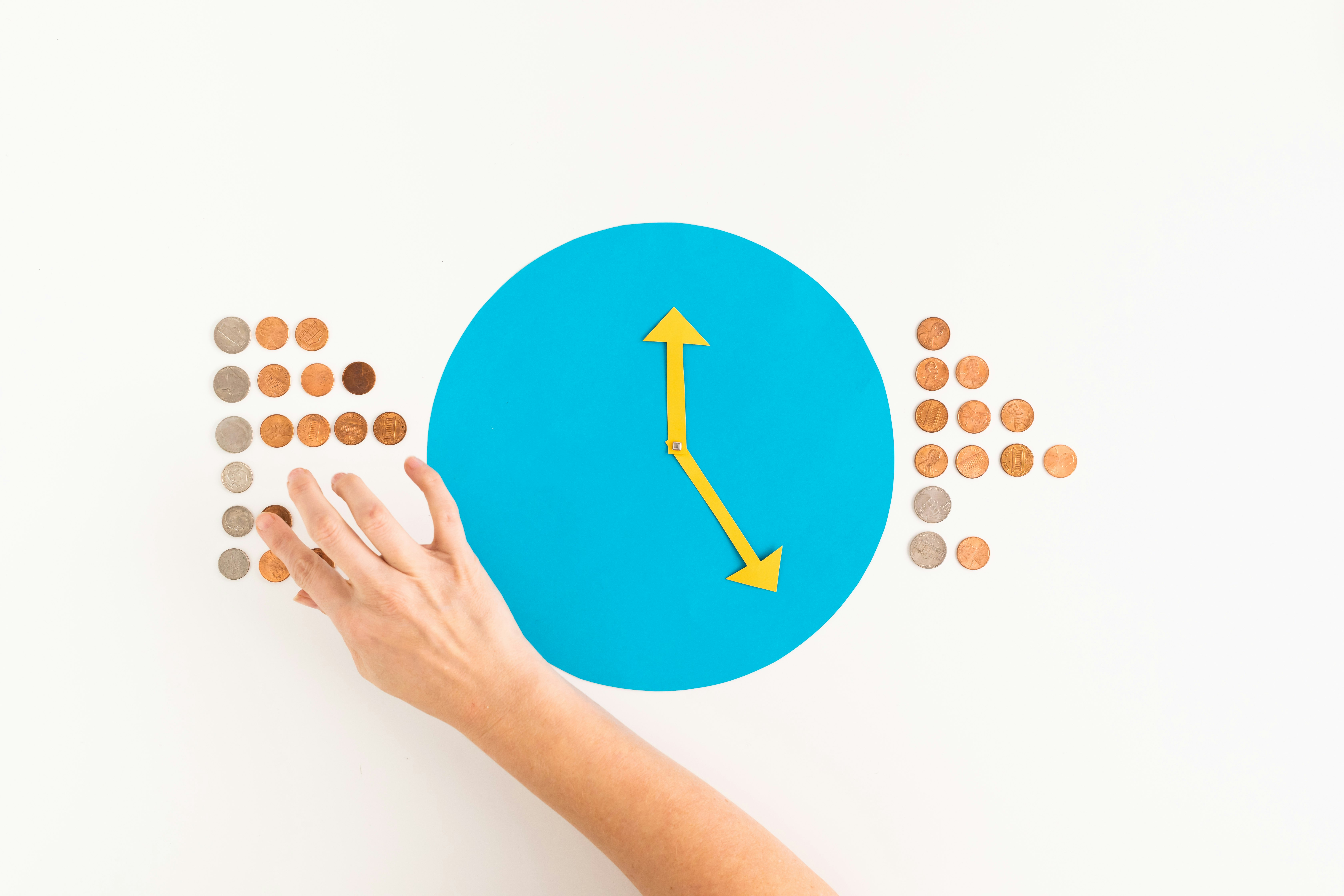• Glue the coins onto the clock using the Elmer’s Extra Strength Glue Stick. (If you’re worried that the glue stick isn’t strong enough to hold the coins, don’t worry! It is!)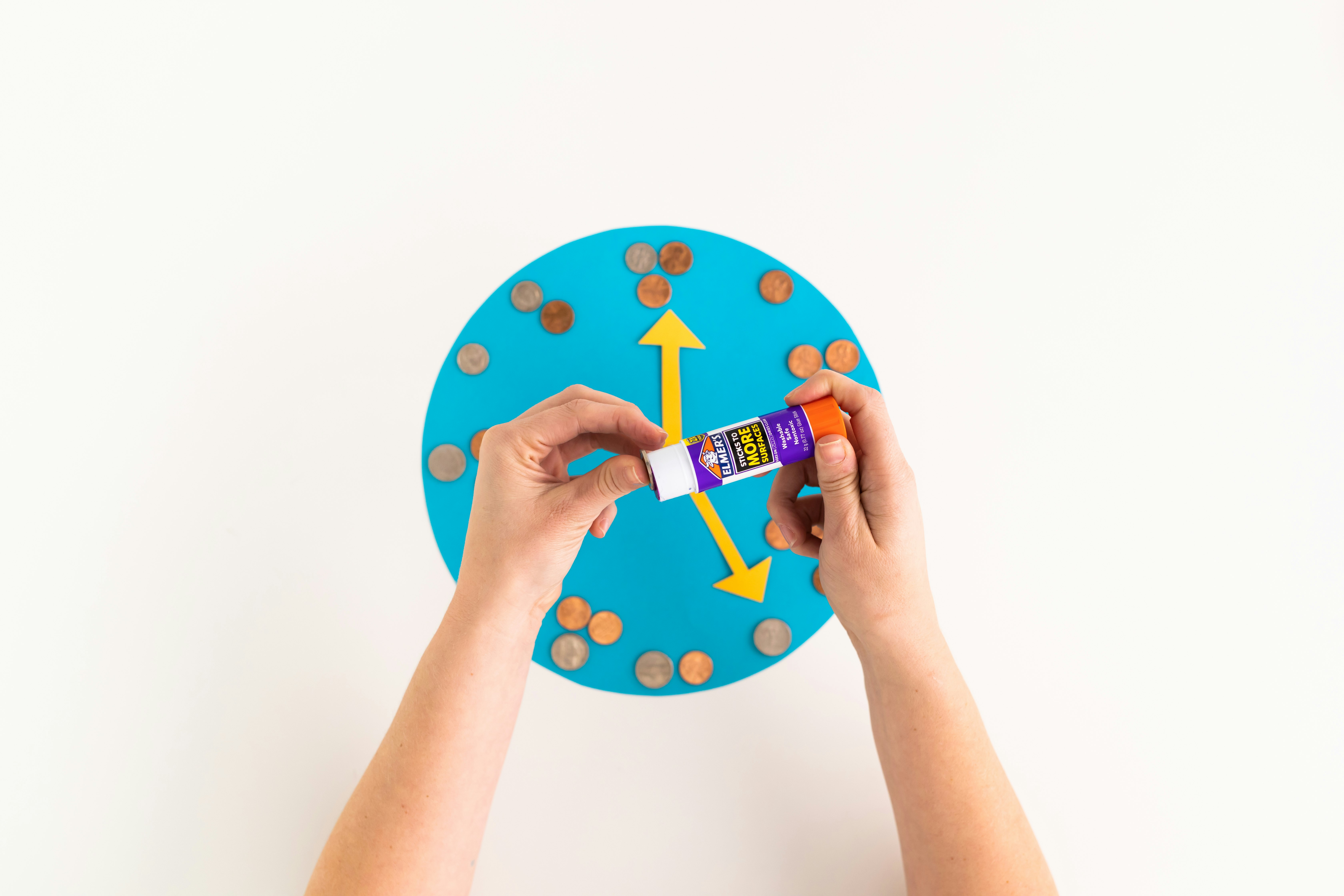• All done! Practice moving the hands of the clock and adding up the coins.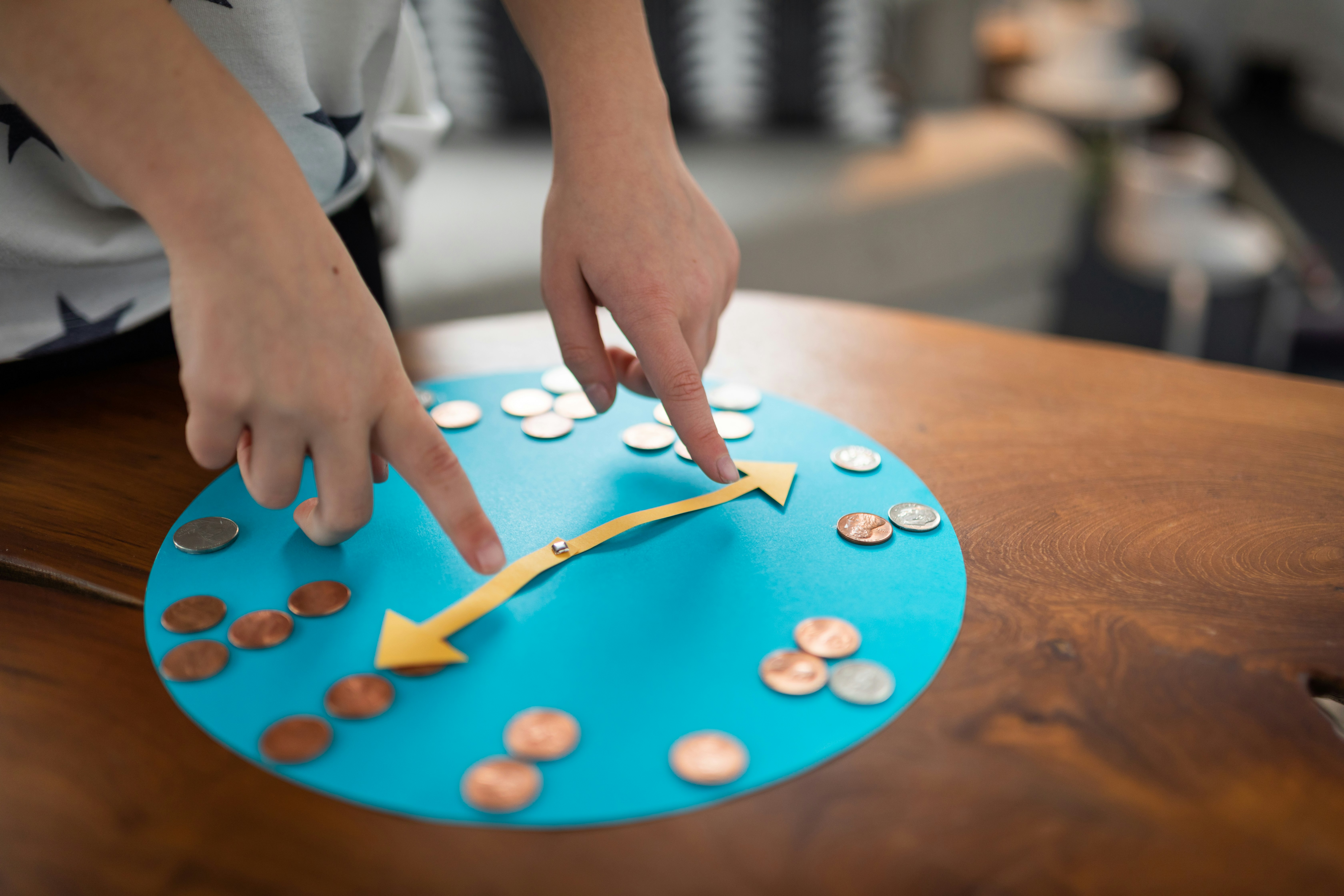• Have you seen a clock like this lately? Possibly the answer is no. Many clocks that we see in the modern world are digital. That means they use numerals to represent the time. The clocks you see in your car, on your appliances, on your cell phone, or on your iPad are all digital clocks. Your coin-counting clock is known as an analog clock. That means the clock has hands to show the minute and hour of the time. The shorter hand indicates the hour, and the longer hand indicates the minute. This type of clock is a bit harder to read than a digital clock, but you will still find analog clocks like this in schools, medical offices, and older buildings. It’s helpful to be able to read both kinds of clocks, so you’re able to easily know the time wherever you are!
• What time is it? Practice using your coin-counting clock to show the time! The short hand goes on the hour. So if it is 3 o’clock, the short hand should be on the 3. Easy peasy, right? Finding where to put the long hand is a little bit trickier. The long hand represents the minute. Since there are 60 minutes in an hour, the long hand moves 60 times every hour. You would have to make your clock a whole lot bigger to place coins to represent every minute, wouldn’t you? To simplify, the larger numbers on your coin clock (represented by the coins) are each worth five minutes (because 60 divided by 12 = 5). So if your long hand is on the 1, then it is representing five minutes. If the long hand is on the four, it represents 20 minutes (which is 4X5). So if it’s 3:25, the short hand is on the 3, and the long hand is on the 5 (which is 5X5). See if you can figure out how to make the following times on your clock:
• 5:20
• 10:15
• 12:30
• 4:45
• How do people tell time? Clocks are not the only way we tell time! There are actually many ways that people tell time. Other common time-telling instruments include watches, marine chronometers, hourglasses, atomic clocks, and sundials. How many other time-telling devices have you used? How many can you find in your house?

Level-Up – Options for Older Kids:

• Coins have possibilities! How many combinations of coins can you use to create the numbers on your clock? Let’s find out. For example, to make the number 10, you could use one dime, but you could also use two nickels. Go around the clock and see how many different combinations of coins you can use to make the numbers.
• Find the centre of the circle. There are lots of methods for finding the centre of a circle, but this one is both fun and easy. Draw a chord across the circle. A chord is a straight line that’s drawn near the edge of the circle but doesn’t cross the centre. Once you have one chord, draw two more chords in any place on the circle that are the exact same length as your first chord. Now you should have three chords, draw a dot on the exact middle of each chord. Then use the end of your ruler to draw a line from each dot that is exactly perpendicular to each chord. Voila! The place where the three lines intersect is the centre of the circle!

Standards Alignment:

Next Generation Science Standards

• CCSS.MATH.CONTENT.1.MD.B.3: Tell and write time - Tell and write time in hours and half-hours using analog and digital clocks.
• CCSS.MATH.CONTENT.2.MD.C.7: Work with time and money - Tell and write time from analog and digital clocks to the nearest five minutes, using a.m. and p.m.
• CCSS.MATH.CONTENT.2.MD.C.8: Solve word problems involving loonies, quarters, dimes, and nickels, using \$ and ¢ symbols appropriately. Example: If you have 2 dimes and 3 nickels, how many cents do you have?